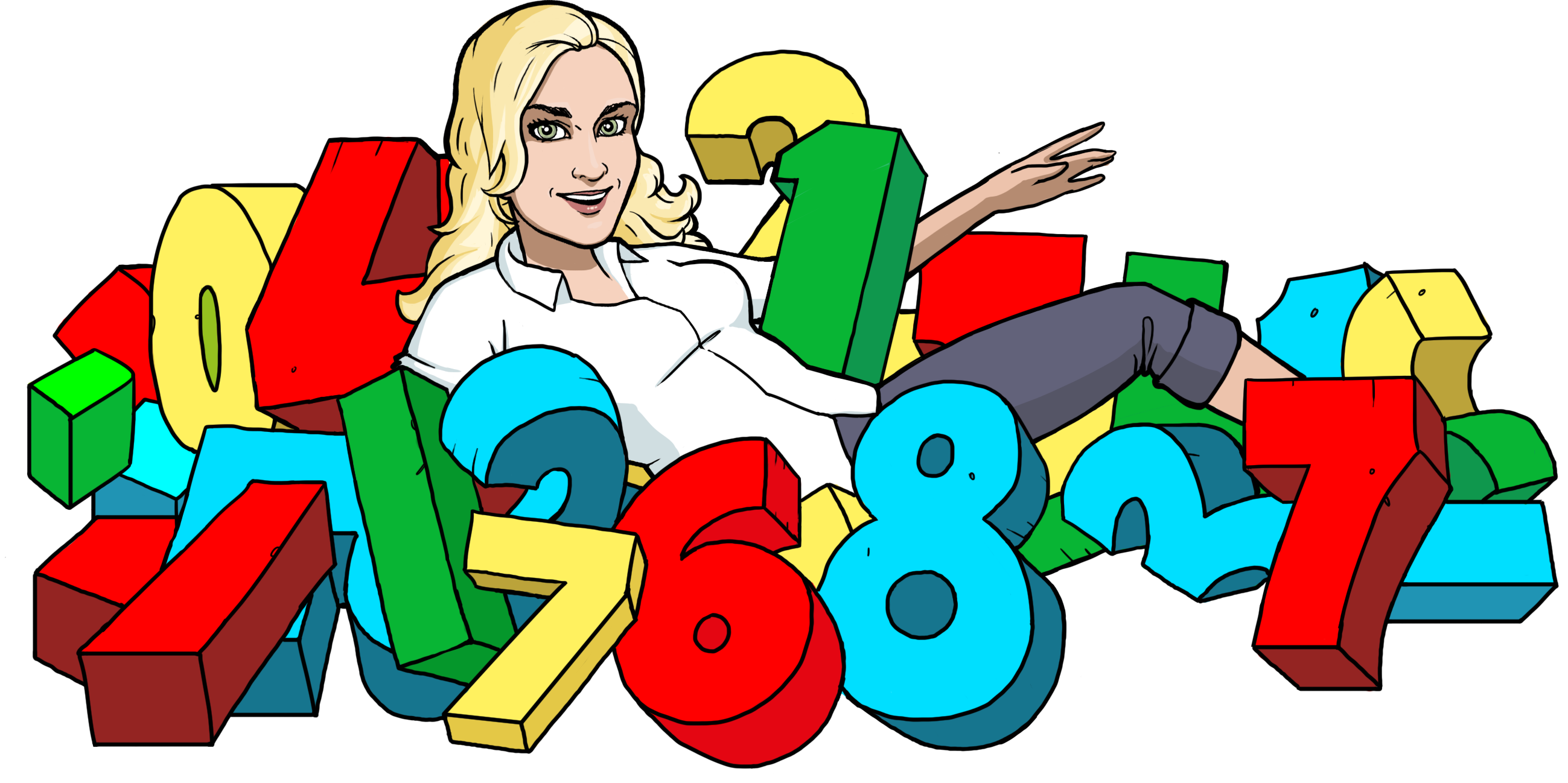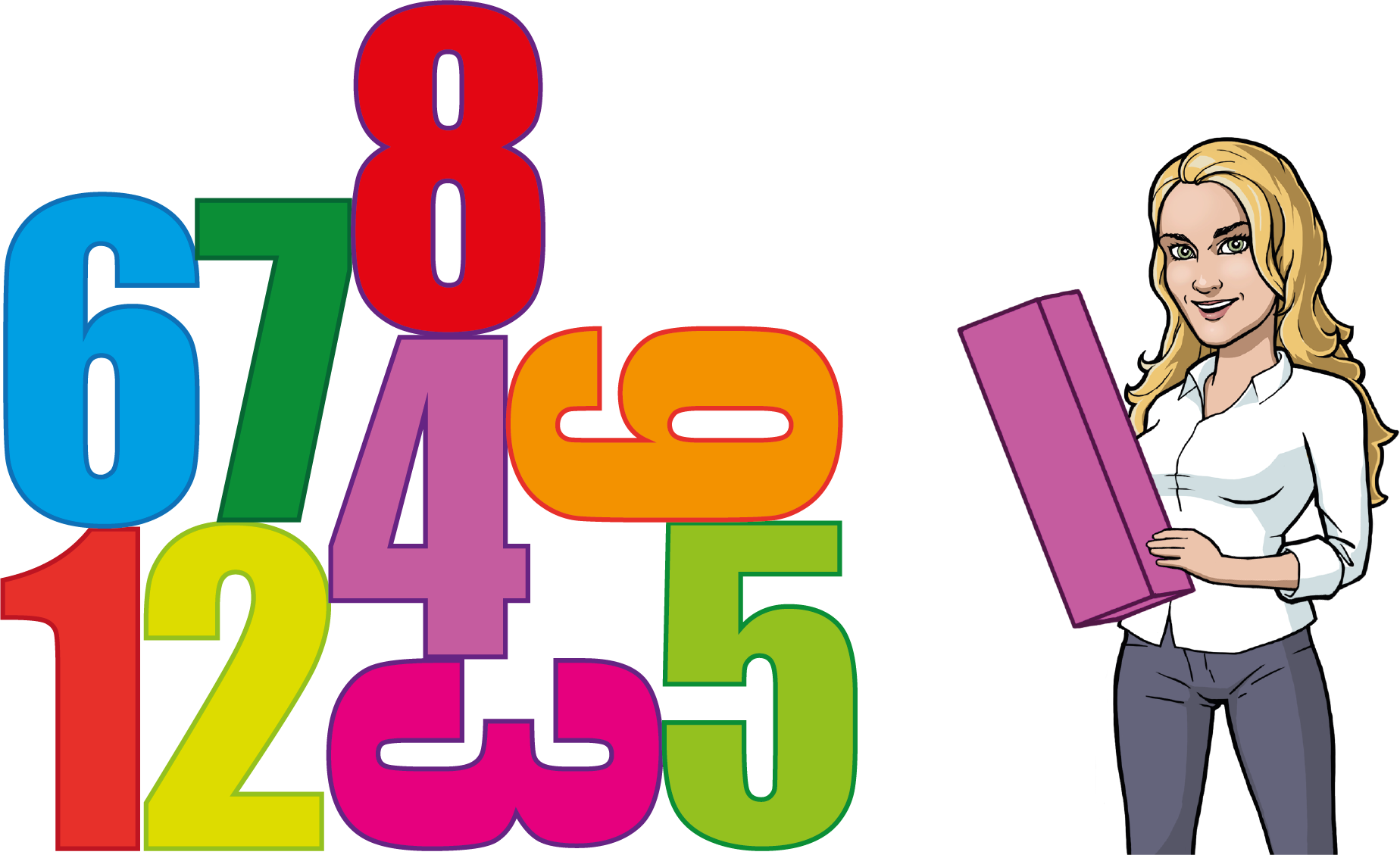Math Topics

# The Digits (0, 1, 2, 3, 4, 5, 6, 7, 8, 9)

A digit is a number that consists of only one symbol. If you think about it, you’ll see that the only numbers like this on the real line are the numbers

 $0,1,2,3,4,5,6,7,8,9.$

All the numbers on the real line are made up of these digits. In short, the ten digits are the building blocks of our numeral system. It might sound a bit strange that these exact numbers can be called both numbers and digits, but it’s no more strange than some of your friends having both a first name and a middle name.There are more numeral systems than just the decimal numeral system. For example, computers and iPads are built on the binary numeral system. This numeral system only use the digits $0$ and $1$.

Can you come up with some numbers and tell me how many digits each number is made up of?

Here are some examples:

• $7$ is made up of one digit,

• $1$ is made up of one digit,

• $-1242$ is made up of four digits,

• $555$ is made up of three digits,

• $283$ is made up of three digits,

• $304$ is made up of three digits,

• $35$ is made up of two digits,

• $-1156$ is made up of four digits,

• $-8991$ is made up of four digits,

• $26$ is made up of two digits,

• $100$ is made up of three digits,

• $68$ is made up of two digits,

• $-55\phantom{\rule{0.17em}{0ex}}334$ is made up of five digits,

• $17\phantom{\rule{0.17em}{0ex}}021$ is made up of five digits,

• $4$ is made up of one digit,

• $534\phantom{\rule{0.17em}{0ex}}564$ is made up of six digits,

• $0$ is made up of one digit,

• $-1\phantom{\rule{0.17em}{0ex}}000\phantom{\rule{0.17em}{0ex}}000$ is made up of seven digits.Math Vault

Would you like to solve exercises about the digits? Try Math Vault!